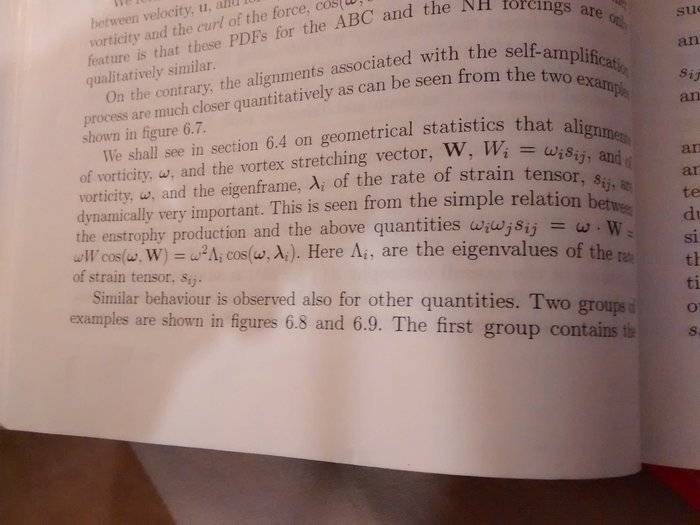• A
What is an eigenframe?andrewkirk
Homework Helper
Gold Member
I've never heard of an Eigenframe and neither, apparently, has Google or DuckDuckGo.

However, there's a fairly natural guess we can make. If a linear operator L on a n-dimensional vector space V is non-degenerate, it will have n orthonormal eigenvectors. These form a nice orthonormal basis for V, and bases can be called 'frames'.

If we are concerned with a differentiable manifold rather than just a single vector space then a (1 1) tensor field T on the manifold can be interpreted as a field of linear operators on the tangent bundle. There will be a unique coordinate frame field whose coordinate directions at any point are those of the eigenvectors of the tensor (qua linear operator) at that point. It would make sense to call that coordinate frame field an 'eigenframe' pf the tensor field T.

I've never heard of an Eigenframe and neither, apparently, has Google or DuckDuckGo.

However, there's a fairly natural guess we can make. If a linear operator L on a n-dimensional vector space V is non-degenerate, it will have n orthonormal eigenvectors. These form a nice orthonormal basis for V, and bases can be called 'frames'.

If we are concerned with a differentiable manifold rather than just a single vector space then a (1 1) tensor field T on the manifold can be interpreted as a field of linear operators on the tangent bundle. There will be a unique coordinate frame field whose coordinate directions at any point are those of the eigenvectors of the tensor (qua linear operator) at that point. It would make sense to call that coordinate frame field an 'eigenframe' pf the tensor field T.

I don't understand any of this.

I've found a few occurrences, however no definition. Maybe you could read more out of its applications than I can.

Density matrices:

Spin:
http://easyspin.org/documentation/hamiltonian.html

https://en.wikipedia.org/wiki/Axiality_and_rhombicity

I don't understand anything on these pages but I think the last link may be onto something but I don't understand the applications part.

I don't understand any of this.

If you don't understand "any of this," which includes eigenvectors, then you haven't studied linear algebra enough to have the prerequisites for tensor analysis.

Also, after you learn linear algebra, you might want to study a more general introductory fluid mechanics text before going back to this one which specializes in turbulence.

•jim mcnamara
If you don't understand "any of this," which includes eigenvectors, then you haven't studied linear algebra enough to have the prerequisites for tensor analysis.

Also, after you learn linear algebra, you might want to study a more general introductory fluid mechanics text before going back to this one which specializes in turbulence.

I understand the physics of what is being explained but wanted it or firmer mathematical background. Just for your reference, engineers don't learn about tangent bundles. Nor do engineers learn much (or anything) about manifolds.

Secondly, you don't know anything about turbulence if indeed your inferring that I need an understanding of tangent bundles and manifolds to understand some of it. If you don't want to help, then fine, but don't be so rude. Please don't post here again, thanks.

fresh_42
Mentor
2021 Award
Eigenvalues and eigenvectors are nothing mysterious. For a linear operator ##L## (function, mapping whatever, as long as it is linear), the equation ##L(x) = Lx = c \cdot x## for a number ##c## means: ##x## is an eigenvector of ##L## with eigenvalue ##c##. That's it. It becomes more interesting when we try to interpret what an eigenvector physically means, or if there is a whole basis consisting of only eigenvectors (to possibly a couple of different eigenvalues).

I think the most likely meaning is, considering what @andrewkirk has said and what is written in the examples I've found, that an eigenframe is either a reference frame that consists of a basis of eigenvectors or more simply: the reference frame of a (moving) particle, since "eigen" means "belonging to", "its property" or "proper". I tend to the latter.

Because "proper" isn't a good translation it has been dropped and people just used the German prefix eigen. (Somewhere here on PF there is a whole thread about the origin and usage of "eigen".)